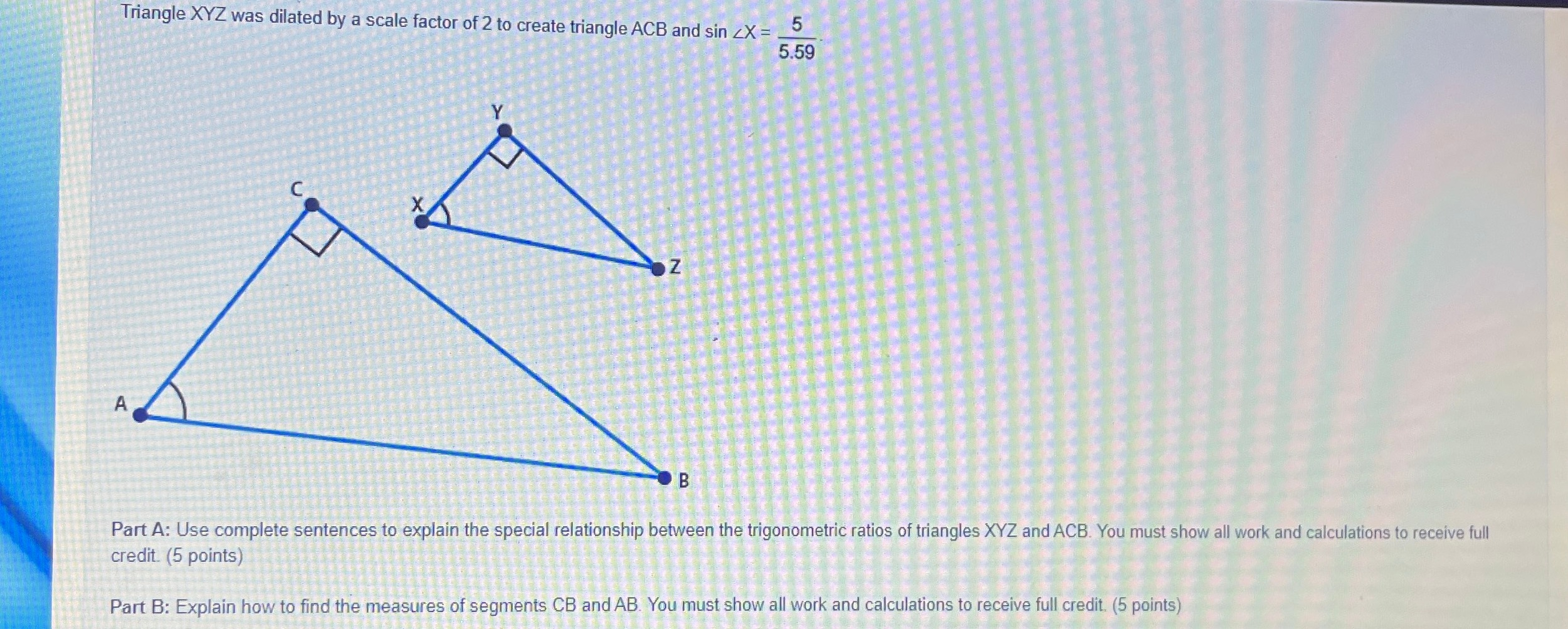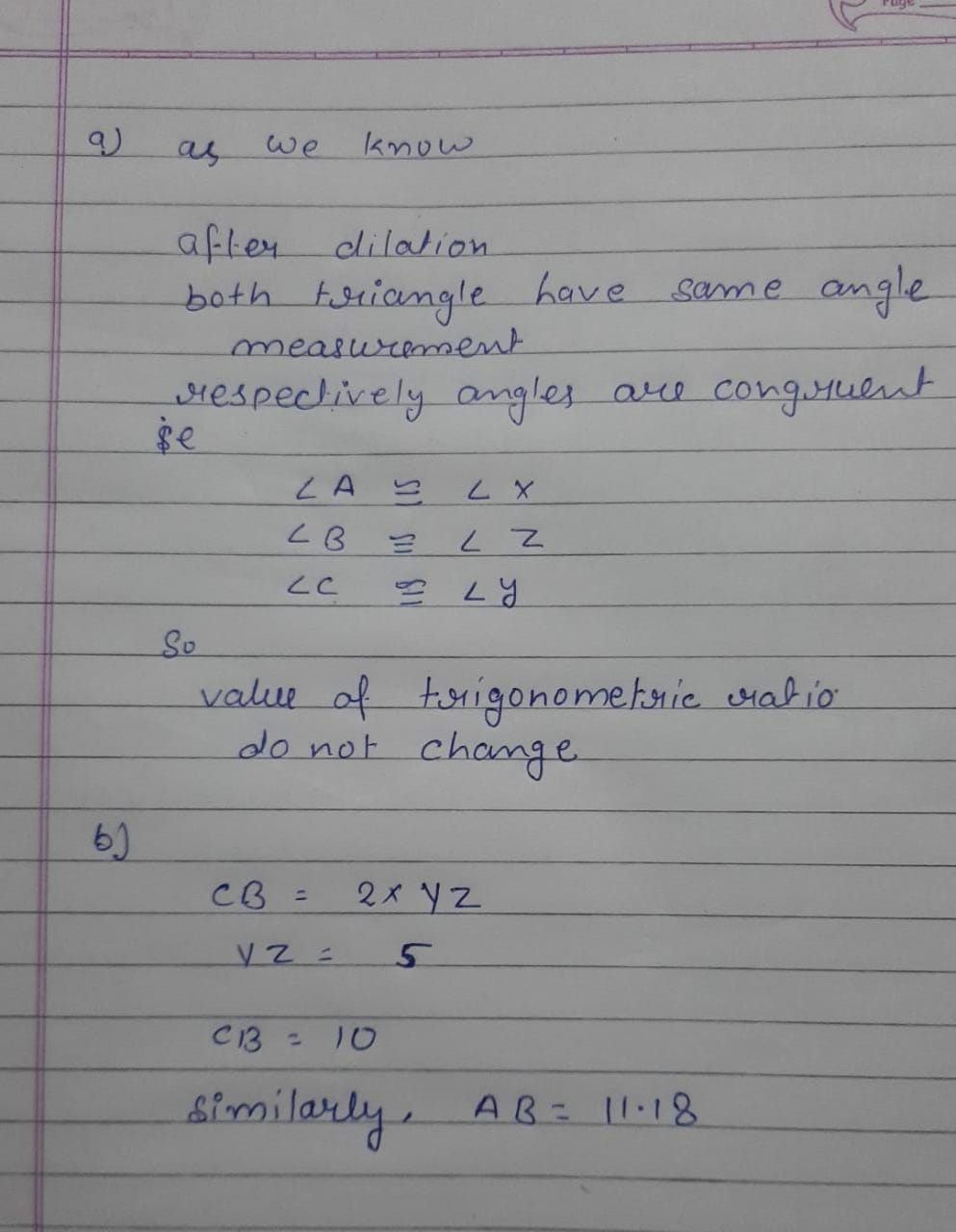### Still have math questions?Triangle XYZ was dilated by a scale factor of $$2$$ to create triangle $$A C B$$ and $$\sin \angle X = \frac { 5 } { 5.59 }$$ . Part A: Use complete sentences to explain the special relationship between the trigonometric ratios of triangles $$X Y Z$$ and ACB. You must show all work and calculations to receive full credit. ( $$5$$ points)